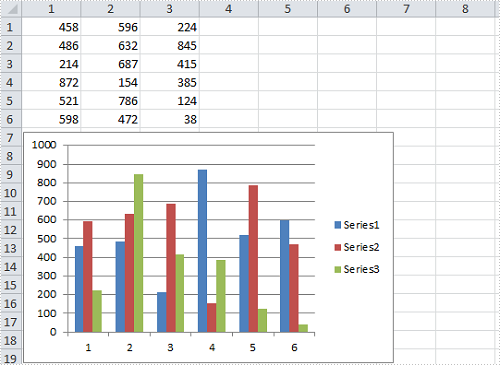# How to Resize and Move Excel Charts in C#, VB.NET

Sometimes, we create a spreadsheet that contains a wonderful chart, we may still want to adjust the size and position of the chart in order to make the chart mostly matches the Excel page. In this article, I'll introduce you how to resize a chart to a suitable scale and how to move a chart to a desired position in C#, VB.NET via Spire.XLS.

Within the class of Spire.Xls.Chart, we can set the parameters of XlsShape.LeftColum and XlsShape.TopRow to move a chart to any location on a worksheet, while the size of the chart can be changed by setting the parameters of XlsShape.Width and XlsShape.Height. More details would be as follows:

Test File:#### Code Snippet for Resize and Move Chart

Step 1: Create a new instance of workbook and load the test file.

```Workbook workbook = new Workbook();
```

Step 2: Get the chart from the first worksheet.

```Worksheet sheet = workbook.Worksheets;
Chart chart = sheet.Charts;
```

Step 3: Set position of the chart.

```chart.LeftColumn = 1;
chart.TopRow = 7;
```

Step 4: Resize the chart.

```chart.Width = 400;
chart.Height = 250;
```

Step 5: Save the changes to a new file.

```workbook.SaveToFile("result.xlsx", ExcelVersion.Version2010);
```

Result:Full Code:

[C#]
```using Spire.Xls;
namespace ResizeandMoveExcel
{
class Program
{

static void Main(string[] args)
{

Workbook workbook = new Workbook();

Worksheet sheet = workbook.Worksheets;
Chart chart = sheet.Charts;

chart.LeftColumn = 1;
chart.TopRow = 7;

chart.Width = 400;
chart.Height = 250;

workbook.SaveToFile("result.xlsx", ExcelVersion.Version2010);
}
}
}
```
[VB.NET]
```Imports Spire.Xls
Namespace ResizeandMoveExcel
Class Program

Private Shared Sub Main(args As String())

Dim workbook As New Workbook()

Dim sheet As Worksheet = workbook.Worksheets(0)
Dim chart As Chart = sheet.Charts(0)

chart.LeftColumn = 1
chart.TopRow = 7

chart.Width = 400
chart.Height = 250

workbook.SaveToFile("result.xlsx", ExcelVersion.Version2010)
End Sub
End Class
End Namespace
```